### Home > MC1 > Chapter 4 > Lesson 4.3.4 > Problem4-122

4-122.
1. . Use a variable expression to represent each sequence of lengths shown below. Homework Help ✎

1.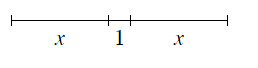2.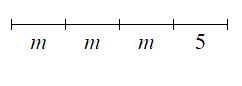3.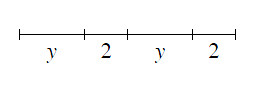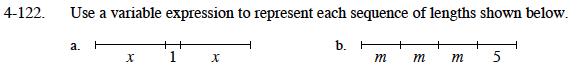Think of this as being a tightrope for Cecil to cross. How would you describe his path using this picture?

2x + 1 or x + 1 + x

This problem is very similar to part (a).
If the lengths labeled ''m'' were instead labeled as ''1'', how would you write the expression?

3m + 5 or m + m + m + 5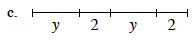Use parts (a) and (b) as guides if you are having trouble.
Remember that the variable represents a number, so you
can use it in an expression just as you would a number.